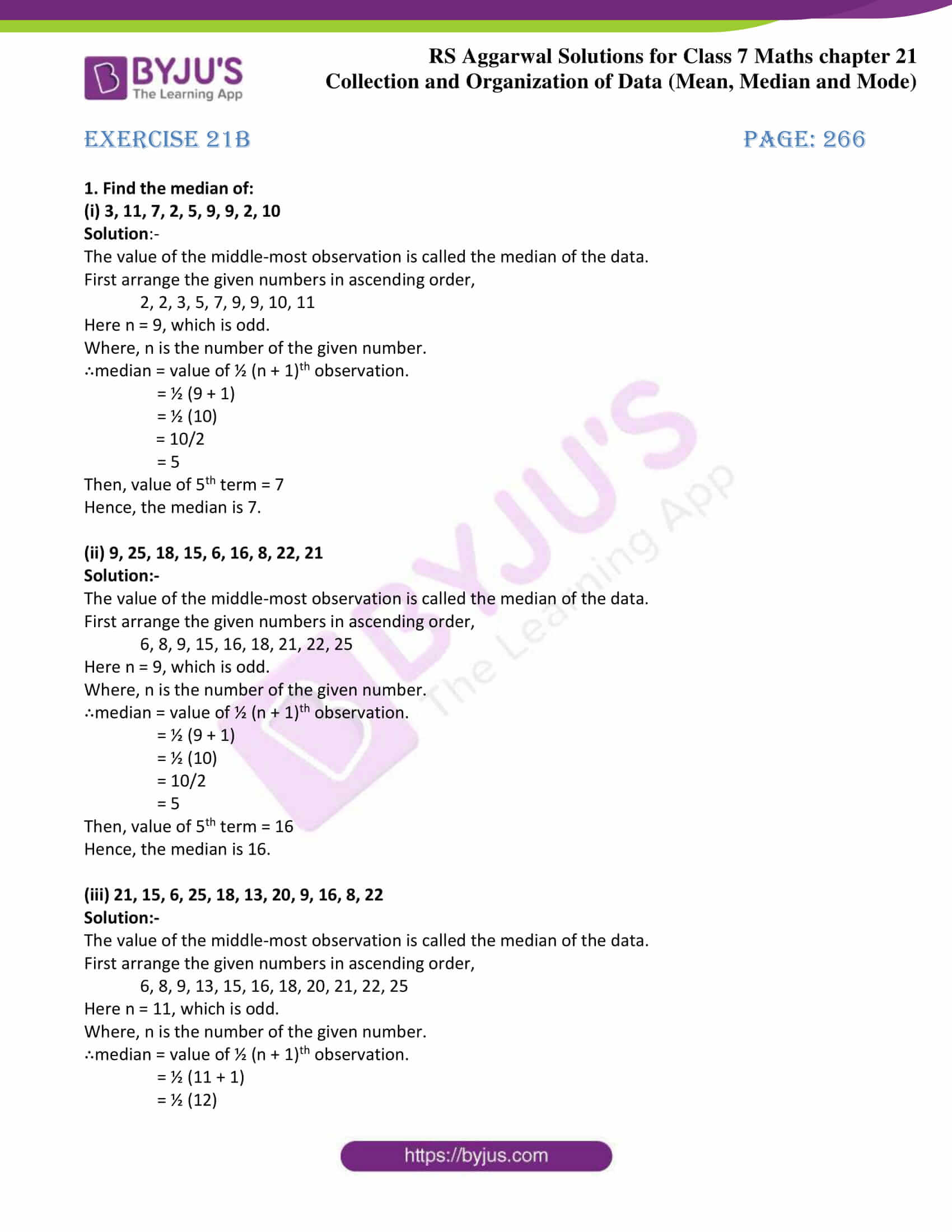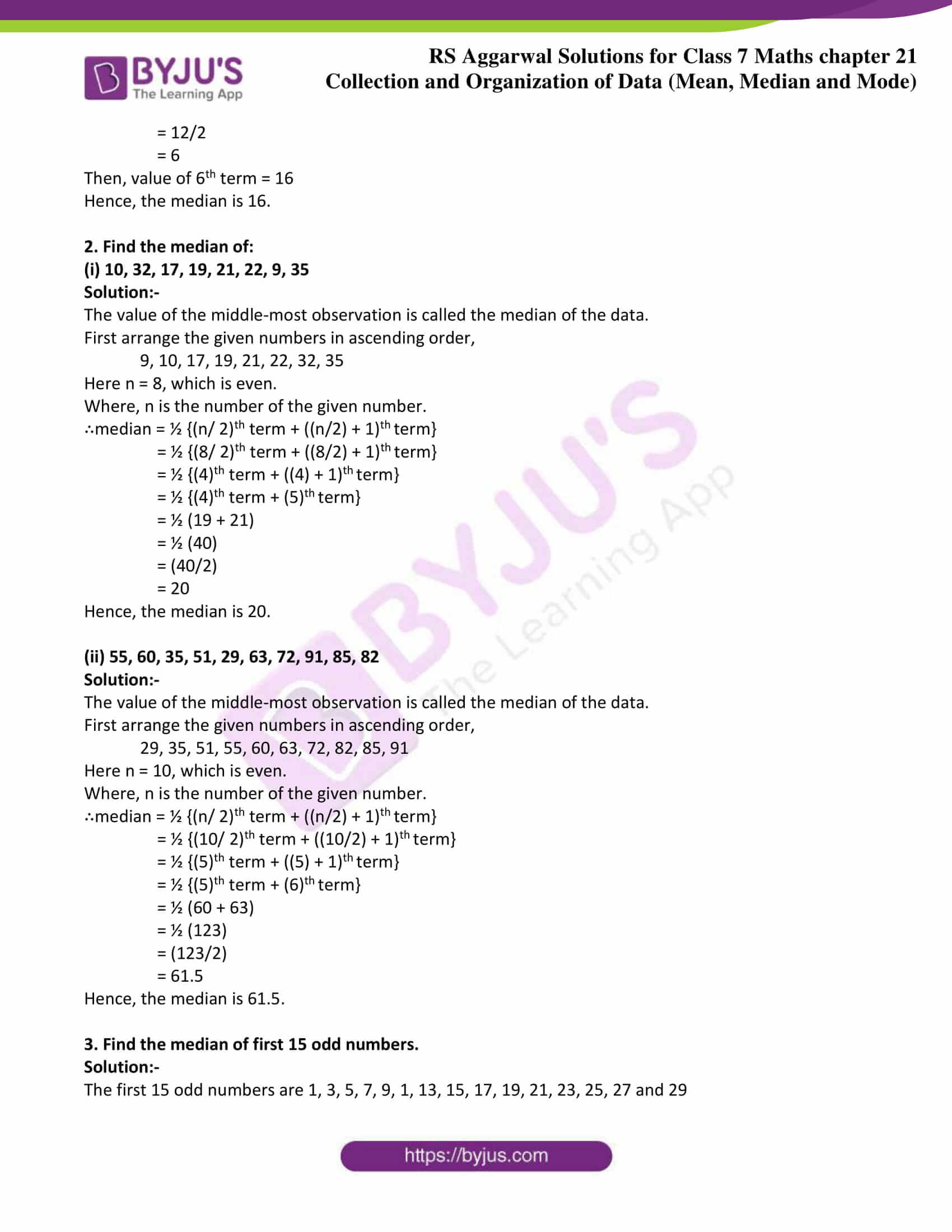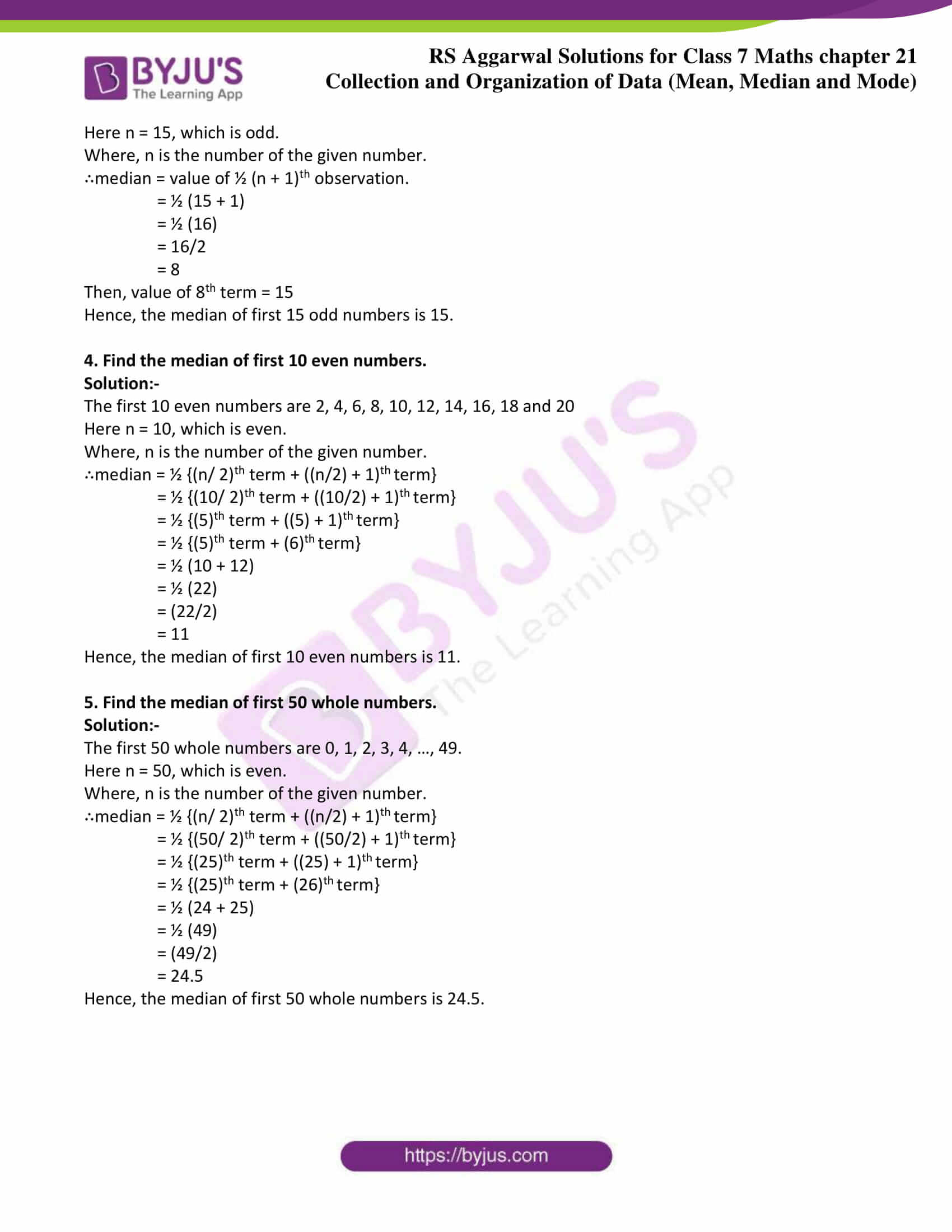# RS Aggarwal Solutions for Class 7 Maths Exercise 21B Chapter 21 Collection and Organisation of Data (Mean, Median and Mode)

The simple PDF of RS Aggarwal Solutions for the Exercise 21B of Class 7 Maths Chapter 21 Collection and Organisation of Data (Mean, Median and Mode) are given here. This exercise of RS Aggarwal Solutions for Class 7 Chapter 21 contains questions related to the median of ungrouped data and median of discrete series. After arranging the given data in ascending or descending order of magnitude, the value of the middle-most observation is the median of the data. RS Aggarwal Solutions for Class 7 Chapter 21 Exercise 21B is prepared by our expert specialist to assist students in a better way, with the help of solutions provided here.

## Download the PDF of RS Aggarwal Solutions For Class 7 Maths Chapter 21 Collection and Organisation of Data (Mean, Median and Mode) – Exercise 21B### Access answers to Maths RS Aggarwal Solutions for Class 7 Chapter 21 – Collection and Organisation of Data (Mean, Median and Mode) Exercise 21B

1. Find the median of:

(i) 3, 11, 7, 2, 5, 9, 9, 2, 10

Solution:-

The value of the middle-most observation is called the median of the data.

First arrange the given numbers in ascending order,

2, 2, 3, 5, 7, 9, 9, 10, 11

Here n = 9, which is odd.

Where, n is the number of the given number.

∴median = value of ½ (n + 1)th observation.

= ½ (9 + 1)

= ½ (10)

= 10/2

= 5

Then, value of 5th term = 7

Hence, the median is 7.

(ii) 9, 25, 18, 15, 6, 16, 8, 22, 21

Solution:-

The value of the middle-most observation is called the median of the data.

First arrange the given numbers in ascending order,

6, 8, 9, 15, 16, 18, 21, 22, 25

Here n = 9, which is odd.

Where, n is the number of the given number.

∴median = value of ½ (n + 1)th observation.

= ½ (9 + 1)

= ½ (10)

= 10/2

= 5

Then, value of 5th term = 16

Hence, the median is 16.

(iii) 21, 15, 6, 25, 18, 13, 20, 9, 16, 8, 22

Solution:-

The value of the middle-most observation is called the median of the data.

First arrange the given numbers in ascending order,

6, 8, 9, 13, 15, 16, 18, 20, 21, 22, 25

Here n = 11, which is odd.

Where, n is the number of the given number.

∴median = value of ½ (n + 1)th observation.

= ½ (11 + 1)

= ½ (12)

= 12/2

= 6

Then, value of 6th term = 16

Hence, the median is 16.

2. Find the median of:

(i) 10, 32, 17, 19, 21, 22, 9, 35

Solution:-

The value of the middle-most observation is called the median of the data.

First arrange the given numbers in ascending order,

9, 10, 17, 19, 21, 22, 32, 35

Here n = 8, which is even.

Where, n is the number of the given number.

∴median = ½ {(n/ 2)th term + ((n/2) + 1)th term}

= ½ {(8/ 2)th term + ((8/2) + 1)th term}

= ½ {(4)th term + ((4) + 1)th term}

= ½ {(4)th term + (5)th term}

= ½ (19 + 21)

= ½ (40)

= (40/2)

= 20

Hence, the median is 20.

(ii) 55, 60, 35, 51, 29, 63, 72, 91, 85, 82

Solution:-

The value of the middle-most observation is called the median of the data.

First arrange the given numbers in ascending order,

29, 35, 51, 55, 60, 63, 72, 82, 85, 91

Here n = 10, which is even.

Where, n is the number of the given number.

∴median = ½ {(n/ 2)th term + ((n/2) + 1)th term}

= ½ {(10/ 2)th term + ((10/2) + 1)th term}

= ½ {(5)th term + ((5) + 1)th term}

= ½ {(5)th term + (6)th term}

= ½ (60 + 63)

= ½ (123)

= (123/2)

= 61.5

Hence, the median is 61.5.

3. Find the median of first 15 odd numbers.

Solution:-

The first 15 odd numbers are 1, 3, 5, 7, 9, 1, 13, 15, 17, 19, 21, 23, 25, 27 and 29

Here n = 15, which is odd.

Where, n is the number of the given number.

∴median = value of ½ (n + 1)th observation.

= ½ (15 + 1)

= ½ (16)

= 16/2

= 8

Then, value of 8th term = 15

Hence, the median of first 15 odd numbers is 15.

4. Find the median of first 10 even numbers.

Solution:-

The first 10 even numbers are 2, 4, 6, 8, 10, 12, 14, 16, 18 and 20

Here n = 10, which is even.

Where, n is the number of the given number.

∴median = ½ {(n/ 2)th term + ((n/2) + 1)th term}

= ½ {(10/ 2)th term + ((10/2) + 1)th term}

= ½ {(5)th term + ((5) + 1)th term}

= ½ {(5)th term + (6)th term}

= ½ (10 + 12)

= ½ (22)

= (22/2)

= 11

Hence, the median of first 10 even numbers is 11.

5. Find the median of first 50 whole numbers.

Solution:-

The first 50 whole numbers are 0, 1, 2, 3, 4, …, 49.

Here n = 50, which is even.

Where, n is the number of the given number.

∴median = ½ {(n/ 2)th term + ((n/2) + 1)th term}

= ½ {(50/ 2)th term + ((50/2) + 1)th term}

= ½ {(25)th term + ((25) + 1)th term}

= ½ {(25)th term + (26)th term}

= ½ (24 + 25)

= ½ (49)

= (49/2)

= 24.5

Hence, the median of first 50 whole numbers is 24.5.

### Access other exercises of RS Aggarwal Solutions For Class 7 Chapter 21 – Collection and Organisation of Data (Mean, Median and Mode)

Exercise 21A Solutions

Exercise 21C Solutions